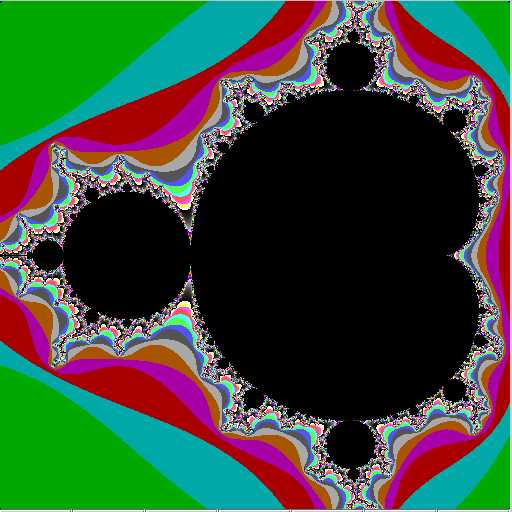# The Mandelbrot Set

Mandelbrot set applet. The following applet allows you to explore the Mandelbrot set by zooming in on different features either by dragging the mouse or entering the desired coordinates. You can also change the color maps to get different cool effects. Requires Java 1.5 or better.

If you don't see a button above, go to java.com and click the Get It Now button.

Mathematical definition. The Mandelbrot set a specific set of points on the plane with many fascinating properties. The points in the Mandelbrot set cannot be described by a single mathematical equation. Instead, to determine whether or not a single point (x, y) is in the Mandelbrot set, we perform the following iterative process.

• Set r0 = x  and   s0 = y.

• Iteratively compute for n = 1 to infinity:

• rn+1 = rn * rn  -  sn * sn  +  x

• sn+1 = 2 * rn * sn  +   y
As an example of this process, the following table shows the first six iterates for the point (x, y) = (0.125, 0.75).

 # 0 1 2 3 4 5 6 r 0.125 -0.421875 -0.575928 0.45501 -0.303564 -1.95998 3.94524 s 0.75 0.9375 -0.041016 0.797244 1.47551 -0.145822 1.32161

If this sequence remains bounded as n goes to infinity (neither rn nor sn goes to infinity) then the point (x, y) is in the Mandelbrot set; otherwise, if the sequence diverges to infinity, it is not. If you plot the points in the Mandelbrot set black, and those not in the set white, a strange and wondrous pattern emerges, roughly within the 2.0-by-2.0 box centered at (-0.5, 0).Plotting on a computer. The mathematical definition of the Mandelbrot set is computationally unappealing, since we would have to perform an infinite number of iterations to determine whether a single point is in the set! We are partially rescued by the following mathematical fact: if r*r + s*s ever gets to be greater than 4, then the point is definitely not in the set. For example, this fact ensures that the point (0.125, 0.75) is not in the set since after 6 iterations the quantity r*r + s*s exceeds 4. There is no simple test that ensures that a point (x, y) is in the set, but if r*r + s*s stays between 0 and 4 during the first 255 iterations (or some other arbitrarily large number), we conclude that point is in the set, although there remains a possibility that we are wrong since it may take more than 255 iterations for our simple test to declare that a point is not in the set.

For the more dramatic color picture above, we replace the white points with color gradations, depending on the number of iterations needed to discover that the point is not in the set. These colors reveal the striking detail of the terrain just outside the Mandelbrot set.

Complex numbers. The mathematical definition of the Mandelbrot is more easily expressed in terms of complex numbers. To determine a single point z = x + y i is in the Mandelbrot set, we perform the following iterative process: set c0 = z and cn+1 = ( cn )2 + z.

Source code. All told, the source code consists of about 750 lines of Java, mostly for the GUI, including blank lines and occasional commments. Feel free to download it all and modify as you see fit. If you put a version on the Web, please include a link back to this site.

High resolution images. I also have publication quality versions of the images above in grayscale and color (4096-by-4096 png, 8192-by-8192 png, and 1500-by-1500 eps) available by request.

Mandelbrot gallery. Here is a gallery of Mandelbrot images.

Kevin Wayne